# Excellent ring

(diff) ← Older revision | Latest revision (diff) | Newer revision → (diff)

A commutative Noetherian ring satisfying the three axioms stated below. It is known that a geometric ring possesses several qualitative properties not inherent in arbitrary Noetherian rings. The concept of an excellent ring makes it possible to take the most important properties of geometric rings axiomatically into account.

Axioms of an excellent ring.

A1. The ringis a universal chain ring. (A ringis said to be a chain ring if for any two prime ideals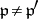of it the lengths of any two saturated chains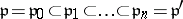of prime ideals are the same. A ringis said to be a universal chain ring if any polynomial ring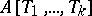is a chain ring.)

A2. The formal fibres ofare geometrically regular, i.e. for any prime ideal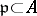and any homomorphism frominto a field, the ring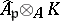is regular. Here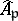is the completion of the local ring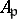.

A3. For any integral finite-algebrathere is a non-zero elementsuch that the ring of fractions,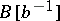, is regular.

Excellent rings possess the following properties:

1) For an excellent ring, the set of regular (normal) points of the scheme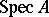is open.

2) If an excellent local ringis reduced (normal or equi-dimensional), then so is the completion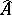.

3) The integral closure of an excellent ringin a finite extension of the field of fractions ofis a finite-algebra.

4) If a ringis excellent, then any-algebra of finite type is also an excellent ring.

Two important examples of excellent rings are the complete local rings (or analytic rings) and the Dedekind rings with field of fractions of characteristic zero. Therefore, the class of excellent rings is sufficiently large and contains, in particular, all algebras of finite type over a field or over the ring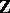of integers.

The excellency of a ringis closely connected with the possibility of resolution of singularities of the scheme(cf.  and ).

How to Cite This Entry:
Excellent ring. Encyclopedia of Mathematics. URL: http://encyclopediaofmath.org/index.php?title=Excellent_ring&oldid=17044
This article was adapted from an original article by V.I. Danilov (originator), which appeared in Encyclopedia of Mathematics - ISBN 1402006098. See original article